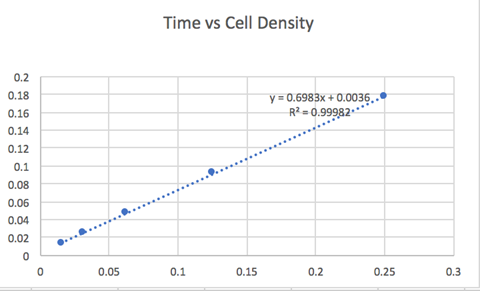TPHS IGEM Wiki

Modeling

# Key Terms

RNA/protein Ratio: This specific ratio is seen in many graphs specifically the Y-Axis. Most of the RNA in E. coli is folded in ribosomes, so they are an integral part of the project. The papers also discuss ribosome synthesis in depth.

Nutrients: The nutrient quality is also very important. There are many equations regarding the nutrient used, and depending on the quality of nutrients over time, the graph becomes linearly or exponentially different.

Main Bacteria used: E. Coli

# Abstract

The goal of this paper is to understand the physiological functions of endogenous genetic circuits. However, in order to do this, we have to study the rate of cell proliferation and the level of gene expression. The main bacteria that they used was E. Coli. The nutrient influx and translation-inhibiting antibiotics seem to play a huge role in this study. Then, we used equations and graphs to model the direction in which mutation will go in.

# Background

Introduction: Engineering of genetic circuits is extremely important. Recent studies show how protein expression and much more can affect the cell’s physiological state. We have to respect growth laws, which provide a framework for the design of robust synthetic systems.

Empirical growth rate dependence: Monod had a discovery between the growth rate of a culture and the concentration of nutrient in the growth medium. In balanced exponential growth, every constituent in the cell is going to double at the same pace. They found this exact same discovery in the work of Salmonella, which is a bacteria in the human body. Similarly, Helmstetter and Cooper studied E. Coli and found that the cell mass has a direct effect on the growth rate. Monod also had very important equations for his growth relation theory. He theorized that the nutritional capacity and the translational capacity can be estimated from the composition of exponentially growing bacteria under the conditions of translation and nutrient limitation.

Quantitative models of bacterial physiology: This part emphasizes growth rate maximization. The growth rate is strongly dependent on many independent factors and many experiments seem to have limitations to them. The growth rate can be altered in many ways, such as ribosome limiting factors and temperature, as these experiments have shown.

Bacterial growth laws: This is where the RNA/Protein ratio take a big part within the experiment. E. Coli is mostly made up of RNA(85%), therefore, the RNA/Protein ratio is directly proportional to the mass fraction of ribosomes in the cell. In terms of nutrient quality, a linear relationship exists between the ribosome content and the growth rate.

 Name Equation Extra equations within original equation Variable Equations Monod’s growth equation λ = λm*[S’/([S]+Km) Λ=λm*(Kn/(Kn+Kt)) λ is the specific growth rate of the microorganisms λm is the maximum specific growth rate of the microorganisms S is the concentration of the limiting substrate for growth Ks is the "half-velocity constant"—the value of S when μ/μmax = 0.5

We use this equation to determine the growth rate of E. coli. This suggests that factors such as the RNA in E. coli affect cell density.

# In-Depth Explanation of the Graph

We plotted the log-log density curve over time and found that there is a linear relationship between the two variables. This correlation shows how we can determine how dense a colony is at a specific time. We tested this by plating E.Coli and measuring the absorbance over time intervals, and we used a series of calculations to find the cell density.# Conclusion

By plotting the specific variables in Monod's equation, we can see that rate is similar to that of the graph above. We suggest that this graphic is extremely useful for determining how dense E. Coli is at a specific time. This can be useful in knowing exactly how much chitin degraded at a specific time point. We use both the equation and the graph to draw quantitative results in error-prone PCR, such as knowing which chitinase plate had the highest efficacy.